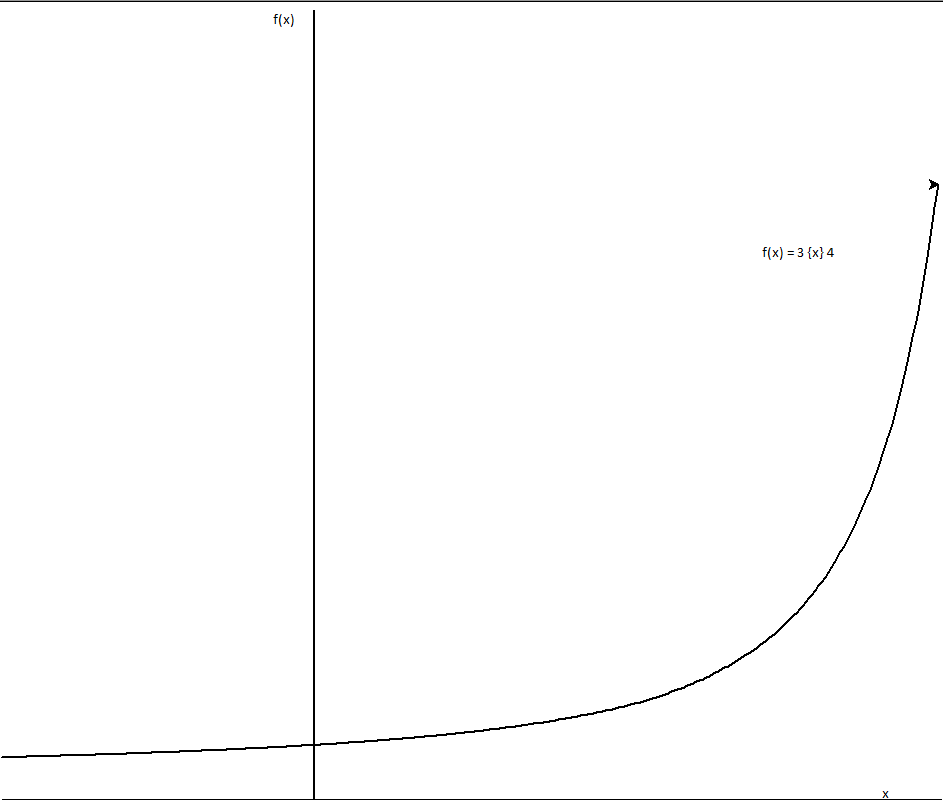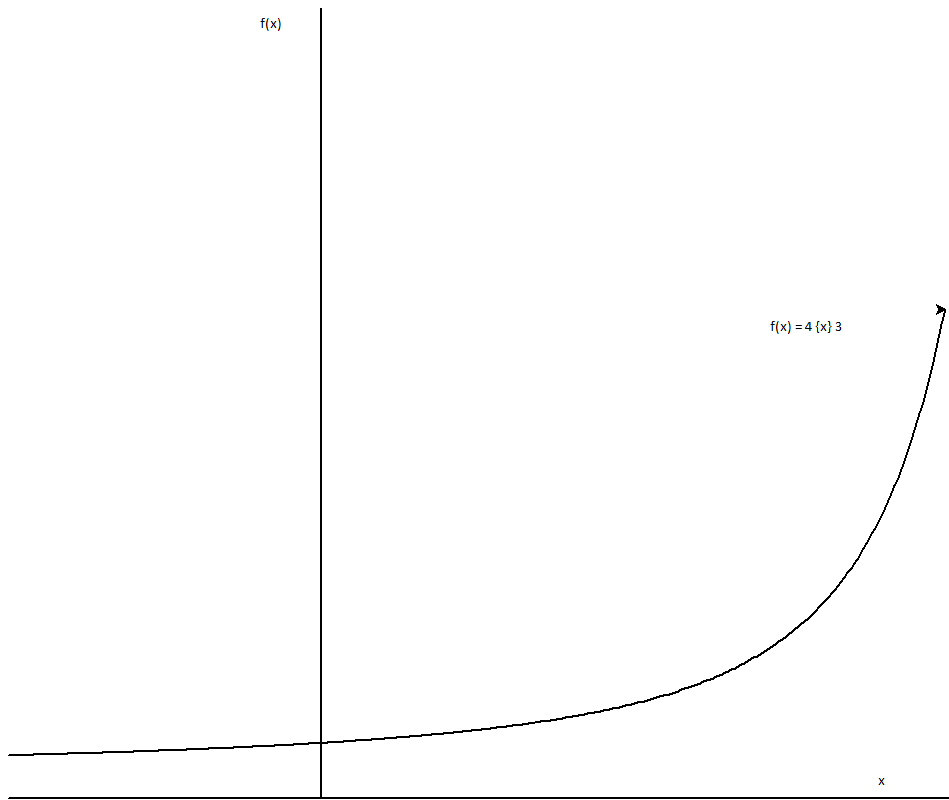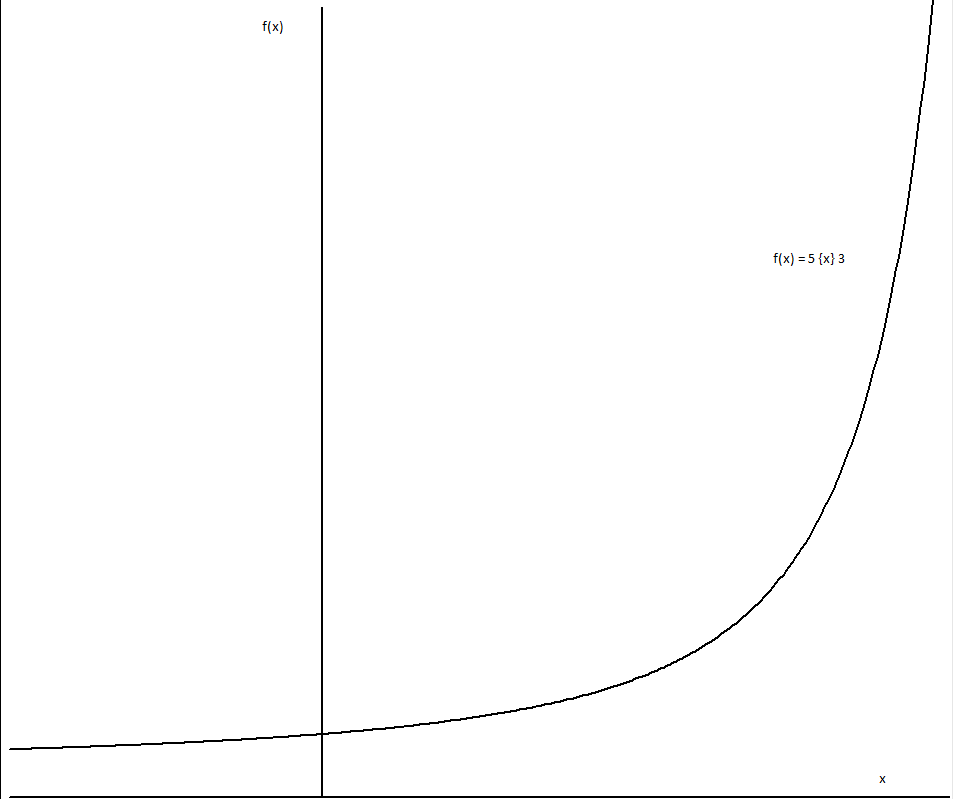• 0 Vote(s) - 0 Average
• 1
• 2
• 3
• 4
• 5
 Rational operators (a {t} b); a,b > e solvedJmsNxnLong Time FellowPosts: 291 Threads: 67 Joined: Dec 2010 06/06/2011, 02:45 AM (This post was last modified: 06/06/2011, 04:42 AM by JmsNxn.) Well alas, logarithmic semi operators have paid off and have given a beautiful smooth curve over domain . This solution for rational operators is given by : Which extends the ackerman function to domain real (given the restrictions provided). the upper superfunction of is used (i.e: the cheta function). Logarithmic semi-operators contain infinite rings and infinite abelian groups. In so far as {t} and {t-1} always form a ring and {t-1} is always an abelian group (therefore any operator greater than {1} is not commutative and is not abelian). There is an identity function S(t), however its values occur below e and are therefore still unknown for operators less than {1} (except at negative integers where it is a variant of infinity (therefore difficult to play with) and at 0 where it is 0). Greater than {1} operators have identity 1. The difficulty is, if we use the lower superfunction of to define values less than e we get a hump in the middle of our transformation from . Therefore we have difficulty in defining an inverse for rational exponentiation. however, we still have a piecewise formula: therefore rational roots, the inverse of rational exponentiation is defined so long as and . rational division and rational subtraction is possible if and . Here are some graphs, I'm sorry about their poor quality but I'm rather new to pari-gp so I don't know how to draw graphs using it. I'm stuck using python right now. Nonetheless here are the graphs. the window for these ones is xmin = -1, xmax = 2, ymin = 0, ymax = 100If there's any transformation someone would like to see specifically, please just ask me. I wanted to do the transformation of as we slowly raise t, but the graph doesn't look too good since x > e. Some numerical values: (I know I'm not supposed to be able to calculate the second one, but that's the power of recursion) I'm very excited by this, I wonder if anyone has any questions comments? for more on rational operators in general, see the identities they follow on this thread http://math.eretrandre.org/tetrationforu...hp?tid=546 thanks, James PS: thanks go to Sheldon for the taylor series approximations of cheta and its inverse which allowed for the calculations.sheldonisonLong Time FellowPosts: 641 Threads: 22 Joined: Oct 2008 06/06/2011, 04:39 AM (This post was last modified: 06/06/2011, 05:54 AM by sheldonison.) (06/06/2011, 02:45 AM)JmsNxn Wrote: Well alas, logarithmic semi operators have paid off and have given a beautiful smooth curve over domain . This solution for rational operators is given by : Which extends the ackerman function to domain real (given the restrictions provided). the upper superfunction of is used (i.e: the cheta function). .....Hey James, Sounds very exciting. fyi, I admit I don't yet understand your new functions, but I made some graphs, and it looks very promising. I thought I'd post the following snippet of pari-gp code, which implements the rational operator function you posted, which can be used with mylatest kneser.gp code, which includes support. This would also make it easier for other to try your new function. If a=1, return (expeta(t-1,b*expeta(1-t,a)))); } I've gotten as far as quickly verifying that for t=0, we have addition, t=1, we have multiplication, and t=2 is exponentiation. The existing code has problems for invcheta(z) or invsexpeta(z), where z is too close to e, and invcheta(z)<=-1000, or invsexpeta(z)>1000. Other than that, it seems to work great. For example, fatb(3,0,4)=7, which is 3+4 fatb(3,1,4)=12, which is 3x4 fatb(3,2,4)=81, which is 3^4 I wonder what it means that fatb(3,-1,4)=5.429897..? Also, is there a smooth continuation to a function for t=3, which would be tetration? - SheldonJmsNxnLong Time FellowPosts: 291 Threads: 67 Joined: Dec 2010 06/06/2011, 05:34 AM (This post was last modified: 06/06/2011, 07:08 AM by JmsNxn.) (06/06/2011, 04:39 AM)sheldonison Wrote: mylatest kneser.gp code, which includes support. This would also make it easier for other to try it. Code:fatb(a,t,b) = {   if (t>=1, return (cheta(invcheta(b*cheta(invcheta(a)+1-t))+t-1)));   if (t<1,  return (cheta(invcheta( cheta(invcheta(a)-t)+cheta(invcheta(b)-t) )+t))); }` Oh that's great! I'd love to see what happens for complex operator values, I'll just modify your code slightly to say real(t) under the if conditions. (06/06/2011, 04:39 AM)sheldonison Wrote: I've gotten as far as quickly verifying that for t=0, we have addition, t=1, we have multiplication, and t=2 is exponentiation. for example, fatb(3,0,4)=7, which is 3+4 fatb(3,1,4)=12, which is 3x4 fatb(3,2,4)=81, which is 3^4 what does it mean that fatb(3,-1,4)=5.429897..? Is there a smooth continuation to a function for t=3, which would be tetration? if ae, and , which would be rational tetration. So actually yes, I can evaluate over domain The formula is given by: And lastly, I tried using sexp/slog base for a,b < e and the graph of is not smooth and there are two humps at about {0.5} and {1.5} which is very unflattering. I was surprised when the cheta function worked, but it did. I have to give myself time to think about it first, but right now I'm sure the extension for a,b <= e is right infront of our eyes. Edit: I edited your code and tested if fatb(3, i, 3) = fatb(3, i+1, 2), and it does. Ackerman function is entering the complex plane me thinks. Edit again: Quote: if a3 or (a  1 = 2) a  1 = a+1 a  1 = a a  1 = a for n>2 As soon as one defines a [t] 1 = a for t > 2, then the whole analytic function is already determined to be a, i.e. it must also be a for t=1 which is wrong. Hence an analytic t |-> a[t]1 will not be constant but somehow meandering between the a's, which is somehow really strange. But I see you gracefully avoided that problem by just defining it for a,b > ePS: 1. , This notation is ambiguous, compare . Please invent a better one! 2. , not \alpha but \circ belongs in the exponent: . This notation is derived from the symbol for function composition .sheldonisonLong Time FellowPosts: 641 Threads: 22 Joined: Oct 2008 06/06/2011, 07:03 AM (06/06/2011, 05:34 AM)JmsNxn Wrote: Oh that's great! I'd love to see what happens for complex operator values, I'll just modify your code slightly to say real(t) under the if conditions....at t=1, which corresponds to multiplication, and is the boundary between the two definitions, it appears continuous in the complex plane (which is quite amazing), but it also looks like the function may not converge there. I tried generating a taylor series for fatb(2,t,3) around t=1, with different samping radii, and different numbers of samping points, and it looks like the derivatives grow without bounds, even though the function is continuous in the complex plane. So far, it appears analytic around t=0.5, or t=1.5. - ShelJmsNxnLong Time FellowPosts: 291 Threads: 67 Joined: Dec 2010 06/06/2011, 08:47 AM (This post was last modified: 06/06/2011, 09:01 AM by JmsNxn.) (06/06/2011, 06:53 AM)bo198214 Wrote: But James, this is not analytic at , if we reformulate: We can say: where is addition and composition of analytic functions, except this one function . The whole function can not be analytic. I wonder why it looks so smooth. I like your definition better--it seems sleeker. I was sort of aware that there was no way I was gonna produce an analytic function over the whole complex domain, I'm happy with analytic in a few regions. Quote:But I see you gracefully avoided that problem by just defining it for a,b > ewell hopefully I'll be having to tackle that problem soon. Quote:PS: 1. , This notation is ambiguous, compare . Please invent a better one! Alright, from henceforth I shall refer to logarithmic semi operators with the following notation: And the inverse is given by: therefore: etc etc.. Quote:2. , not \alpha but \circ belongs in the exponent: . This notation is derived from the symbol for function composition . I knew there was something off about my equations. lol (06/06/2011, 06:02 AM)sheldonison Wrote: Hey James, try my code snippet, which I updated while you were posting. It will work for values of a and b 2... at least I think so.bo198214Administrator Posts: 1,389 Threads: 90 Joined: Aug 2007 06/06/2011, 09:23 AM (06/06/2011, 08:47 AM)JmsNxn Wrote: I was sort of aware that there was no way I was gonna produce an analytic function over the whole complex domain, I'm happy with analytic in a few regions.Well not on the whole complex plane, but on the real axis, wouldnt that be nice? I anyway wonder whether thats possible at all. As is not even differentiable at 1, I wonder whether f is. Did you compare the derivations from left and right? Quote:Alright, from henceforth I shall refer to logarithmic semi operators with the following notation: oh I thought }t{ was kinda the inverse in t. But as I see that it is not ... there are already conventions that we use on the forum, even ASCII capable: a  b = a+ b a  b = a*b a  b = a^b so the operator is [t] (one off from your {t}). But then for the inverse functions b [t] x = y y /[t] x = b b [t]\ y = x for example x / y = x - y x \ y = -x + y x / p is the p-th superroot (which is not equal to x  1/p) b \ x is the superlogarithm to base b. This is also in sync with the convention for quasigroups, i.e. groups with non-associative operation but with left- and right-inverse. There the left- and right inverse are written as / and \.tommy1729Ultimate FellowPosts: 1,372 Threads: 336 Joined: Feb 2009 06/06/2011, 11:59 AM what is the idea or intention behind working with base eta ?nuninho1980FellowPosts: 95 Threads: 6 Joined: Apr 2009 06/06/2011, 05:16 PM (This post was last modified: 06/06/2011, 05:18 PM by nuninho1980.) (06/06/2011, 04:39 AM)sheldonison Wrote: I wonder what it means that fatb(3,-1,4)=5.429897..?no, it isn't correct, sorry. fatb(2,-1,2) = 2º2=4 -> 2+2=4 fatb(3,-1,3) = 3º3=5 -> 3+2=5 fatb(4,-1,3) = 4º3=5 - it's correct. because that's here down: aºb if a>b then a+1 if b>a then b+1 if a=b then a+2 « Next Oldest | Next Newest »

 Possibly Related Threads... Thread Author Replies Views Last Post Thoughts on hyper-operations of rational but non-integer orders? VSO 2 1,161 09/09/2019, 10:38 PM Last Post: tommy1729 Hyper operators in computability theory JmsNxn 5 5,056 02/15/2017, 10:07 PM Last Post: MphLee Recursive formula generating bounded hyper-operators JmsNxn 0 1,822 01/17/2017, 05:10 AM Last Post: JmsNxn holomorphic binary operators over naturals; generalized hyper operators JmsNxn 15 18,710 08/22/2016, 12:19 AM Last Post: JmsNxn The bounded analytic semiHyper-operators JmsNxn 2 4,202 05/27/2016, 04:03 AM Last Post: JmsNxn Bounded Analytic Hyper operators JmsNxn 25 23,836 04/01/2015, 06:09 PM Last Post: MphLee Incredible reduction for Hyper operators JmsNxn 0 2,558 02/13/2014, 06:20 PM Last Post: JmsNxn interpolating the hyper operators JmsNxn 3 5,780 06/07/2013, 09:03 PM Last Post: JmsNxn Number theory and hyper operators JmsNxn 7 8,815 05/29/2013, 09:24 PM Last Post: MphLee Number theoretic formula for hyper operators (-oo, 2] at prime numbers JmsNxn 2 4,543 07/17/2012, 02:12 AM Last Post: JmsNxn

Users browsing this thread: 1 Guest(s)